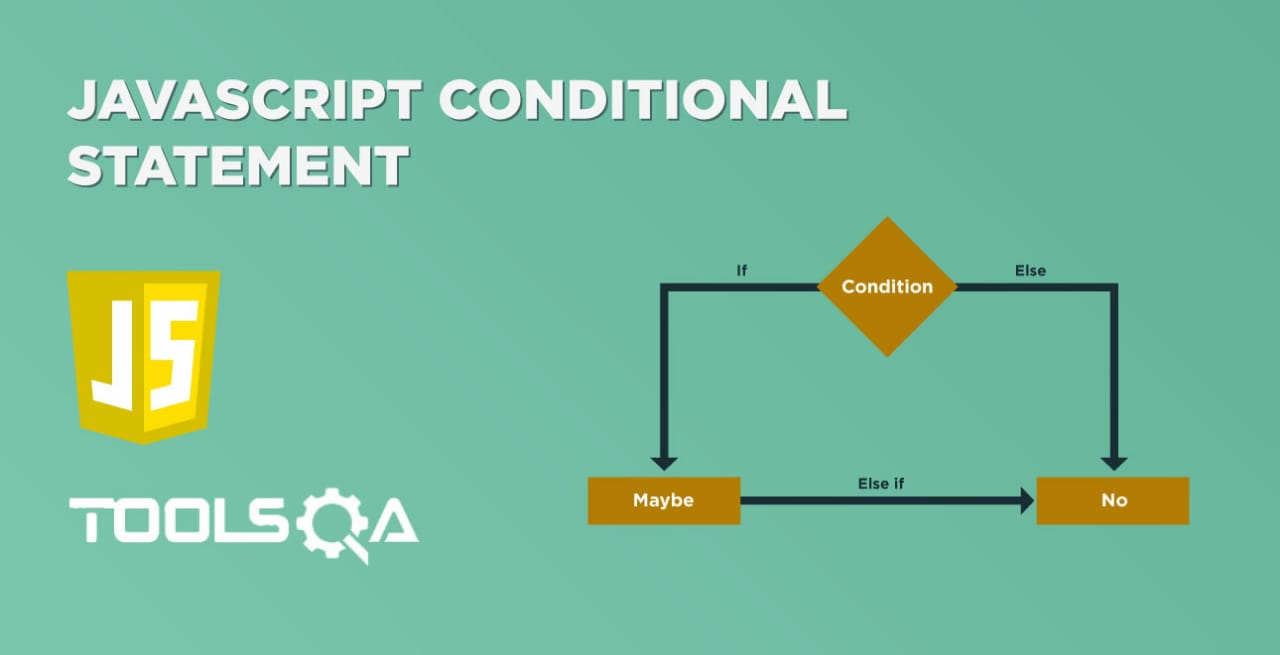In our day to day routine, we have to do multiple tasks repetitively. Suppose we consider a scenario that a user is searching for a word in a dictionary, for which the user needs to go through various pages of the glossary to do the same task. Now, the task that the user is performing is the same - searching the word. But for that, the user needs to repeat the same task on multiple pages to reach the final result. The same is the concept with programming languages, where a particular piece of code needs to execute multiple times to achieve specific tasks. Now to handle these repetitive scenarios, all the programming languages provides the concept of "loops". Let's understand the idea of the loop in JavaScript by going through the following topics in this article:

• What are the loops in programming languages?

• What are the different types of Loops provided by JavaScript?

• How to write For Loop in JavaScript?
• How to write For In Loop in JavaScript?
• Procedure to write For-Of  Loop in JavaScript?
• How to write While Loop in JavaScript?
• How to write Do-While Loop in JavaScript?

## What are the loops in programming languages?

Loops are one of the most fundamental concepts available in all programming languages. The loop will execute the set of code repeatedly until the given condition is satisfied. Loop will ask a question; if the given answer is satisfied, then it will perform some actions, again it will ask a question, and this repeats until no further action required. Each time the question is asked, it is called an iteration. We can depict it with the help of the following diagram:

A programmer who needs to execute the same lines of code, again and again, can use loops. The basic idea behind a loop is to automate the repetitive tasks within a program to save time and effort.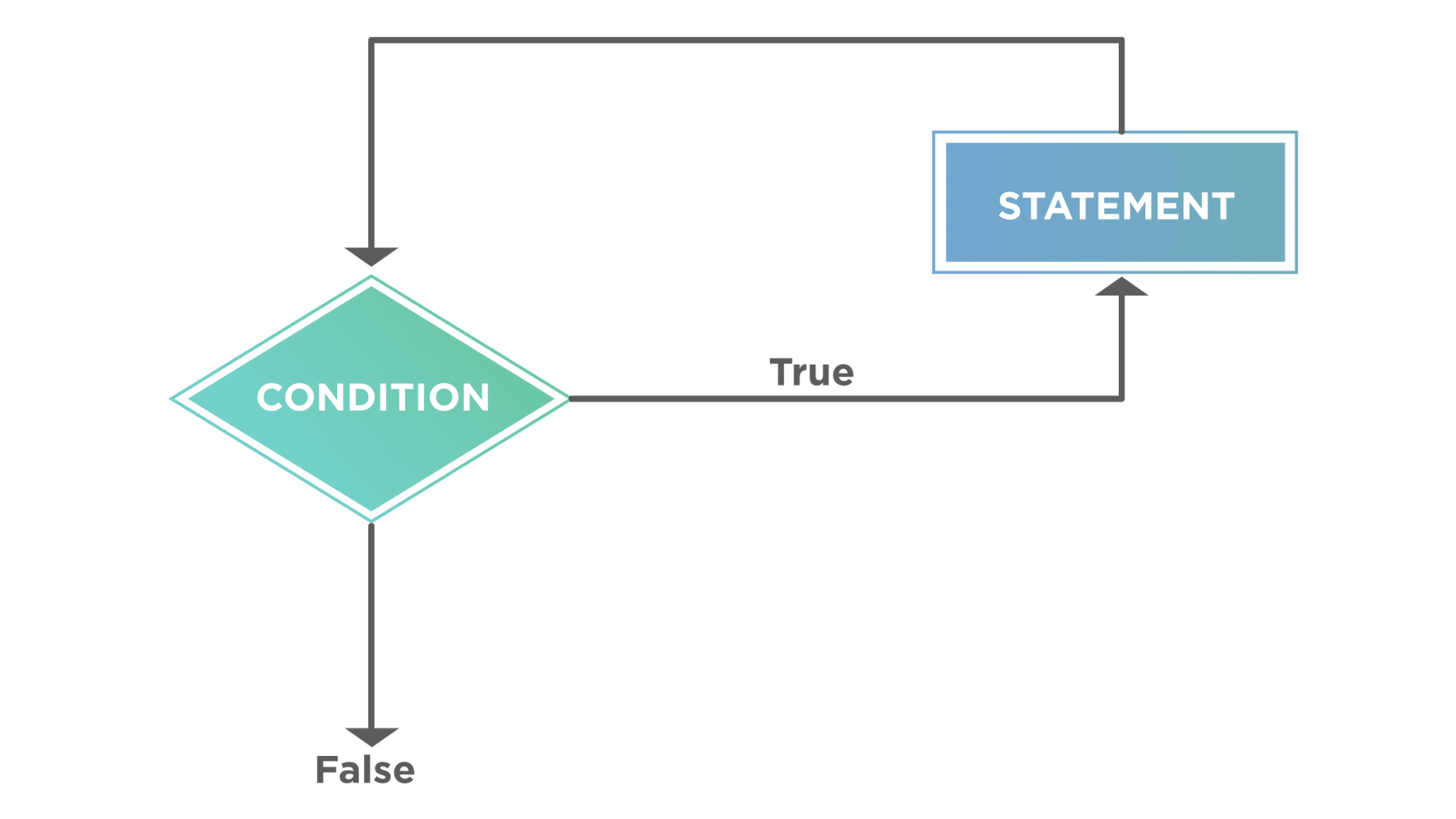## What are the different types of JavaScript Loops?

JavaScript supports five different types of loops, which we will be covering in this article:

• For Loop
• For-In Loop
• For-Of Loop
• While Loop
• Do-While Loop

### How to write For Loop in JavaScript?

A for loop repeats until a specified condition results false. It follows a simple syntax of initializing the initial index, checking the values against a predefined condition, and finally increment/decrement the index until the condition evaluates to false. Its syntax will look like below:

Syntax:

``````for(initialization; condition; increment/decrement){

//Statement need to be executed inside the loop

}
``````

Let's understand all the components of For loop, will help with the following example:1. for is a reserved keyword used to indicate the start of for loop.
2. It is the initialization part where the loop index initializes.
3. Loop will continue to execute until the mentioned condition is satisfied.
4. Increment/decrement of the index variable will happen, which controls the loop.
5. The body of the loop contains logical statements that need to iterate.

Let's deep dive further to understand the working of "for" loop with the help of following code snippet:

``````<html>

<body>

Demonstrating For loop statement

</br>

<script type = "text/javascript">

var n = 5;

var i=0;

for(i=1;i<=n; i++){

document.write(i);

document.write("</br>");

}

document.write("</br>");

</script>

</body>

</html>

``````

Save the file with name forStatement.html and open it in any browser (Chrome, Firefox, or IE). It should show the output as: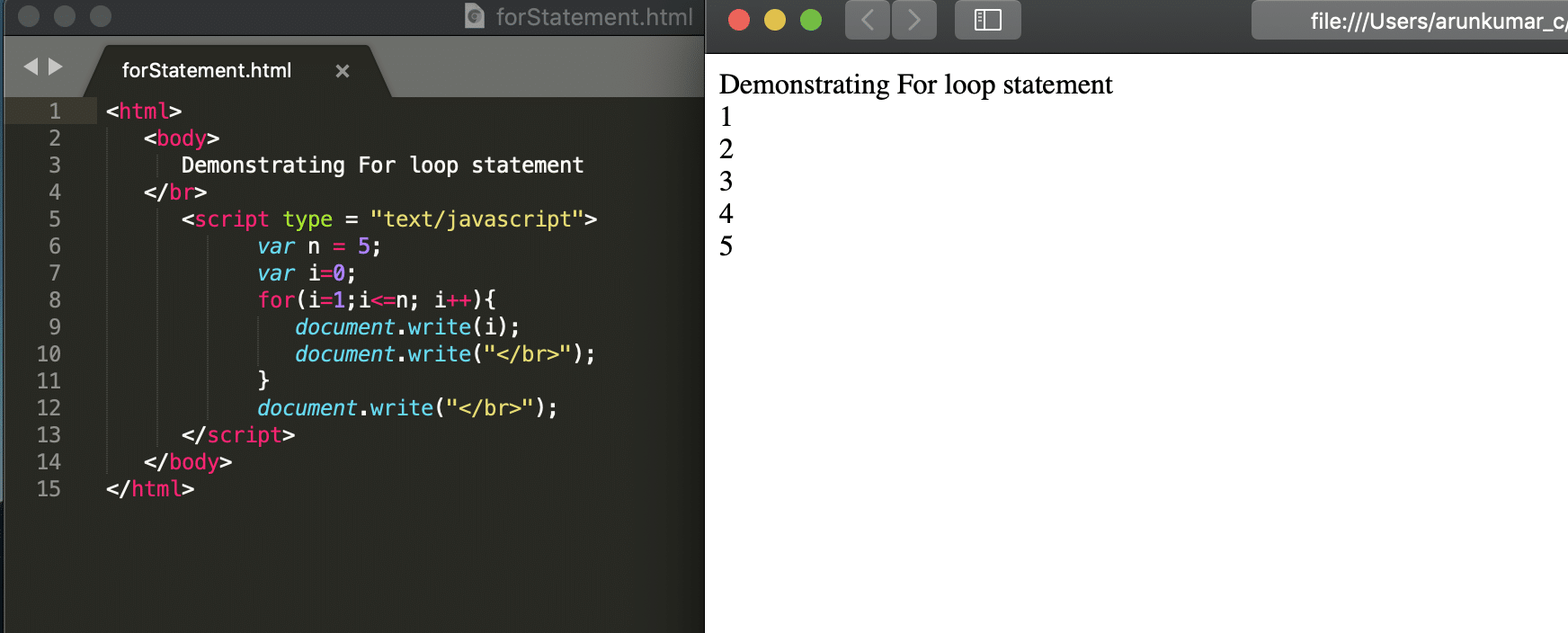As is evident from the above screenshot, the for loop initializes to index 1, and it runs until the value of index "i" reaches to 6. After each iteration, the value of index "i" is incremented by one and then again compared with the condition "i<=n" which will evaluate to true for values of "i" from 1 to 5 but will evaluate to false, once the value of index "i" reaches 6.

Note: the "for" loop is an entry controlled loop, which means that first, the evaluation of the loop condition happens, and then the iteration will start. So, if the condition fails at the start, then the loop will not execute even once.

### How to write For-In Loop in JavaScript?

JavaScript also includes another version of for loop, also known as the for..in Loops. The for..in loop provides a more straightforward way to iterate through the properties of an object. The for...in loop will execute for all the elements in the object, and its syntax will look like below:

Syntax:

``````for ( var in object){

// statement need to be executed;

}
``````

Let's understand all the components of For..in loop, with the help of the following example: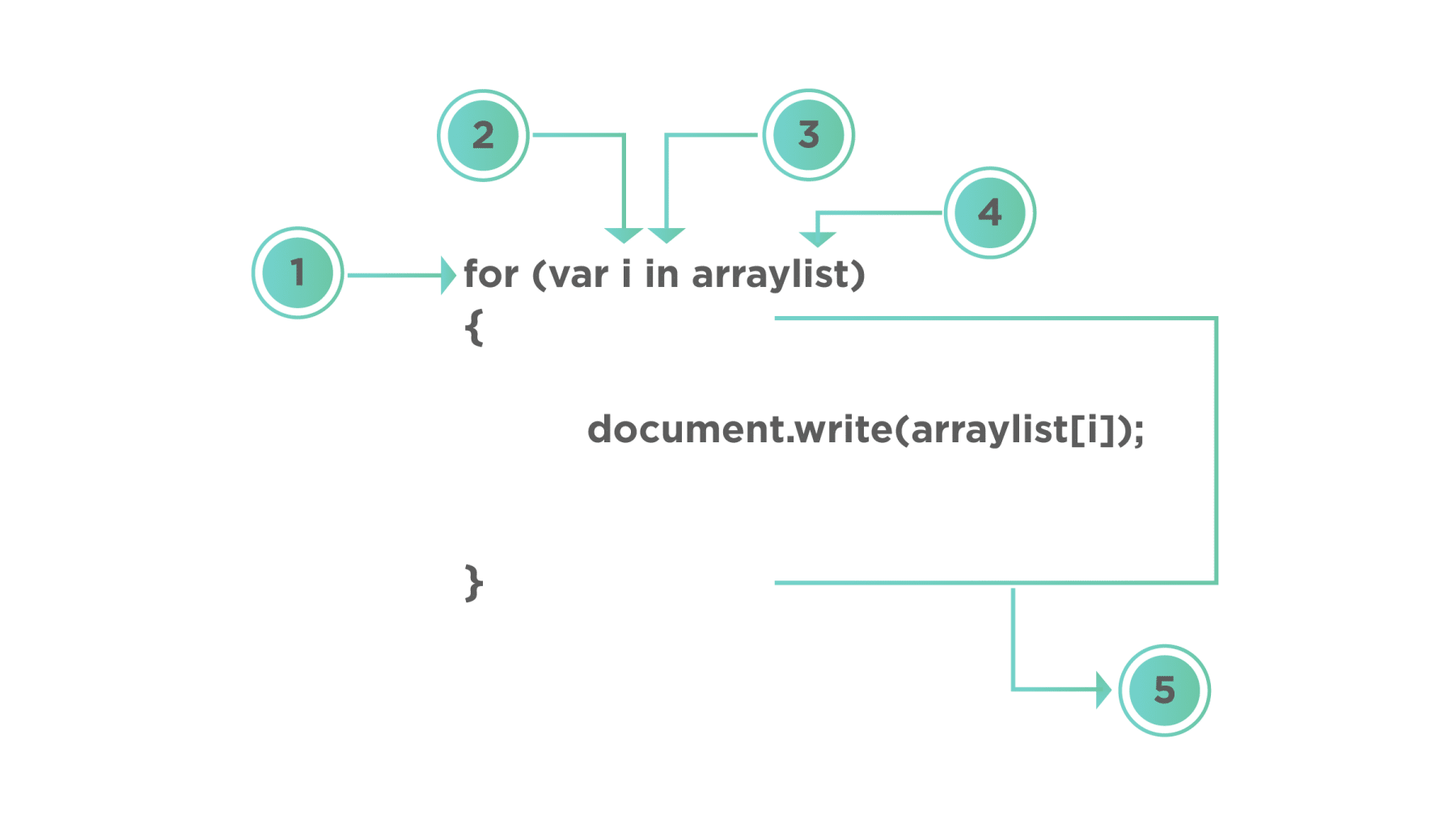1. 'for' is a reserved keyword used to indicate the for loop
2. Declare and initializing the variable to control the loop in this case variable will store only the index value
3. 'in' is a reserved keyword used to indicate a loop for all the elements in the ArrayList
4. ArrayList is the variable that contains array data or list data. We will cover Arrays in the next chapters.
5. The body of the loop contains the logical statements that need to iterate.

Let's understand the working of "for..in" loop with the help of following code snippet:

``````<html>

<body>

Demonstrating for..in loop statement

</br>

<script type = "text/javascript">

var fruits = ['apple', 'grapes', 'orange', 'banana'];

for(const i in fruits){

document.write(i +" => " + fruits[i]);

document.write("</br>");

}

document.write("</br>");

</script>

</body>

</html>

``````

Save the file with name forInStatement.html and open it in any browser (Chrome, Firefox, or IE). It should show the output as: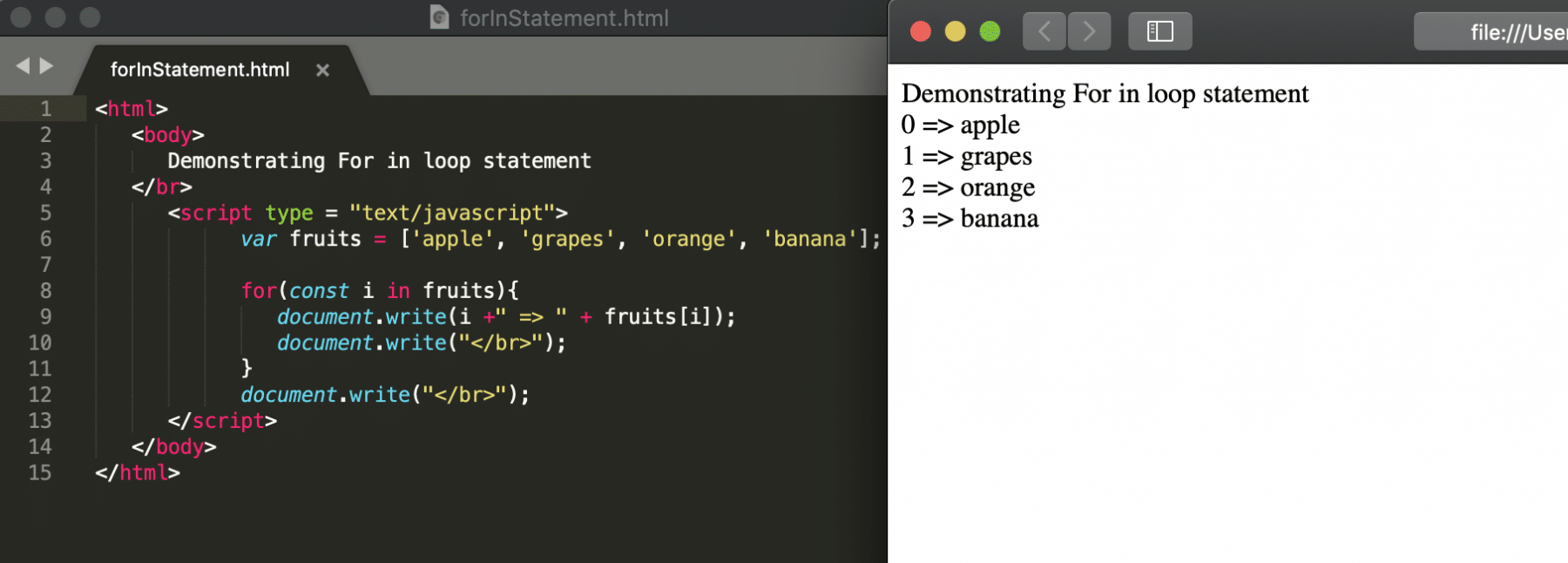In the above example, we have demonstrated the for..in loop. Here, an array of variable fruits has been looped and printed. The variable "i" is storing the index of each element during the iteration.

### How to write For-Of loop in Javascript?

For-of loop is similar to for-in loop except that instead of returning a "key list", it will return the "actual values" of the object. It lets you loop over data structures that are iterable such as Arrays, Strings, Maps, NodeLists, etc. Its syntax will look like below:

Syntax:

``````for (var of object){
//Statements need to be executed.

}
``````

Let's understand all the components of For..of loop, with the help of the following example: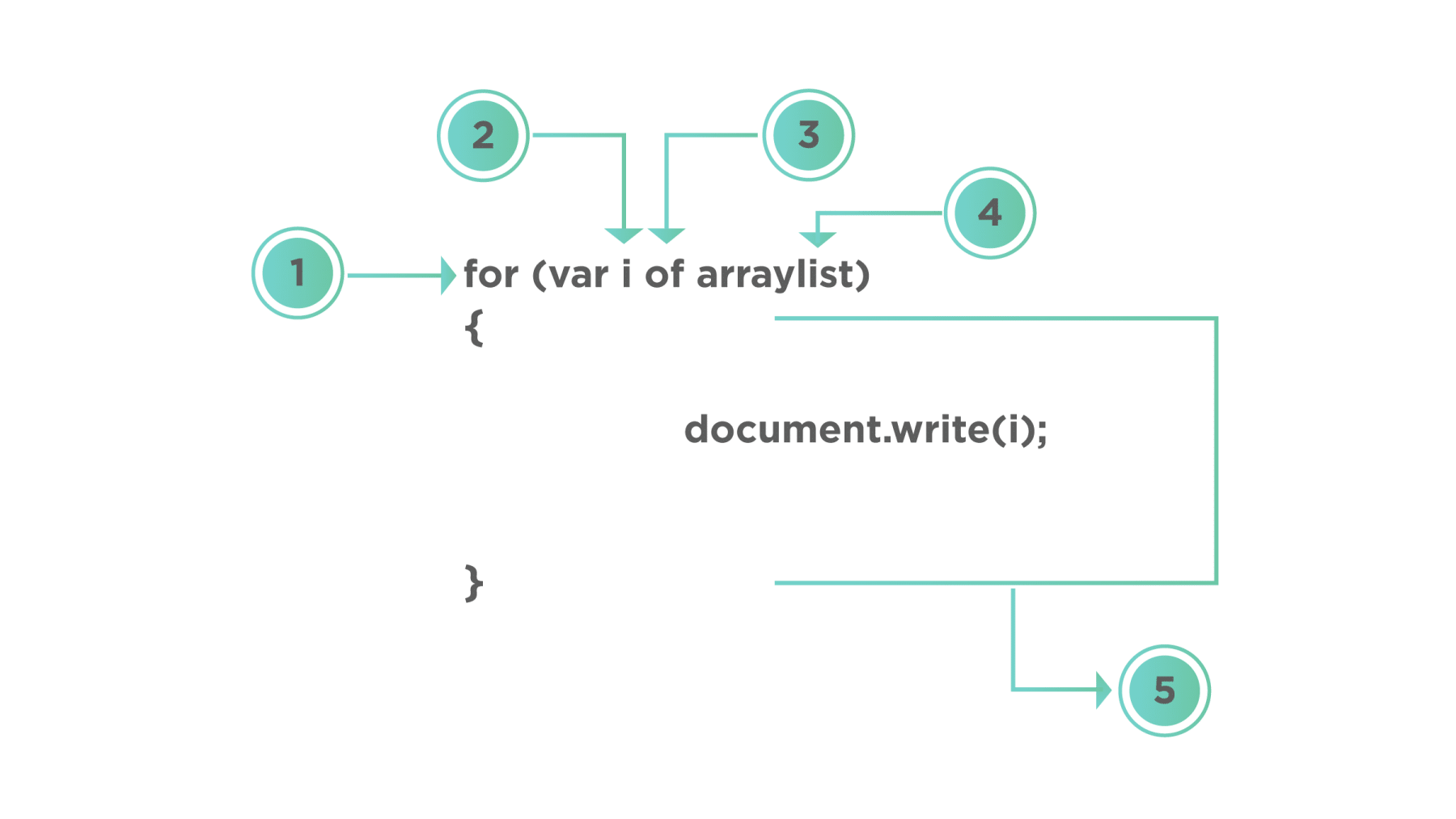1. 'for' is a reserved keyword used to indicate the for loop
2. Declare and initialize the variable to control the loop in this case variable is the actual value of ArrayList on a particular index
3. 'of' is a reserved keyword used to indicate loop for all the elements in the ArrayList
4. ArrayList is the variable which contains array data or list data which we are going to study under Array section
5. Body of the loop where statements need to execute.

Let's understand the working of "for..of" loop with the help of following code snippet:

``````<html>

<body>

Demonstrating for..of loop statement

</br>

<script type = "text/javascript">

var fruits = ['apple', 'grapes', 'orange', 'banana'];

for(const i of fruits){

document.write(i);

document.write("</br>");

}

document.write("</br>");

</script>

</body>

</html>

``````

Save the file with name forOfStatement.html and open it in any browser (Chrome, Firefox, or IE). It should show the output as:In the above example, we have demonstrated a for-of loop where an array of variable fruits is looped and printed. The variable "i" is storing the value of each element during the iteration.

### While Loop in Javascript

The "While" loop, loops through a block of code as long as a specified condition is true. The following flowchart illustrates the "while"  loop statement: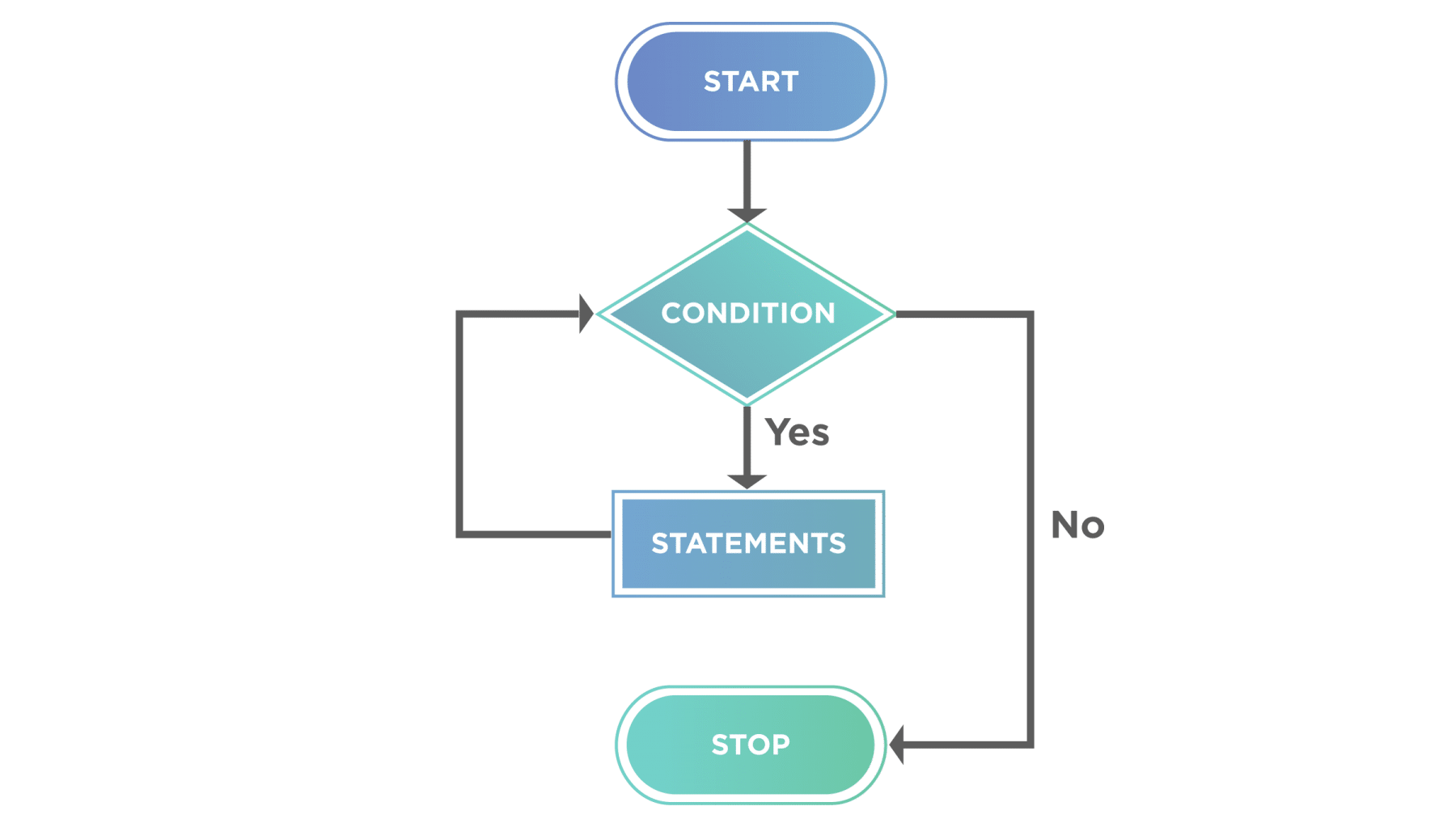Here we can see that the statements will execute until the condition is true. Also, the evaluation of the condition happens first, and then the statements will execute, which makes it an "entry-controlled" loop.

The syntax of the "while" loop looks like below:

Syntax:

``````while(condition){
//Statments need to get executed
}
``````

Let's understand the working of "while" loop with the help of following code snippet:

``````<html>

<body>

Demonstrating While loop statement

</br>

<script type = "text/javascript">

var num  = 10

var i =1;

while(i<=num){

document.write(i);

document.write("</br>");

i++;

}

document.write("</br>");

</script>

</body>

</html>
``````

Save the file with name whileStatement.html and open it in any browser (Chrome, Firefox, or IE). It should show the output as :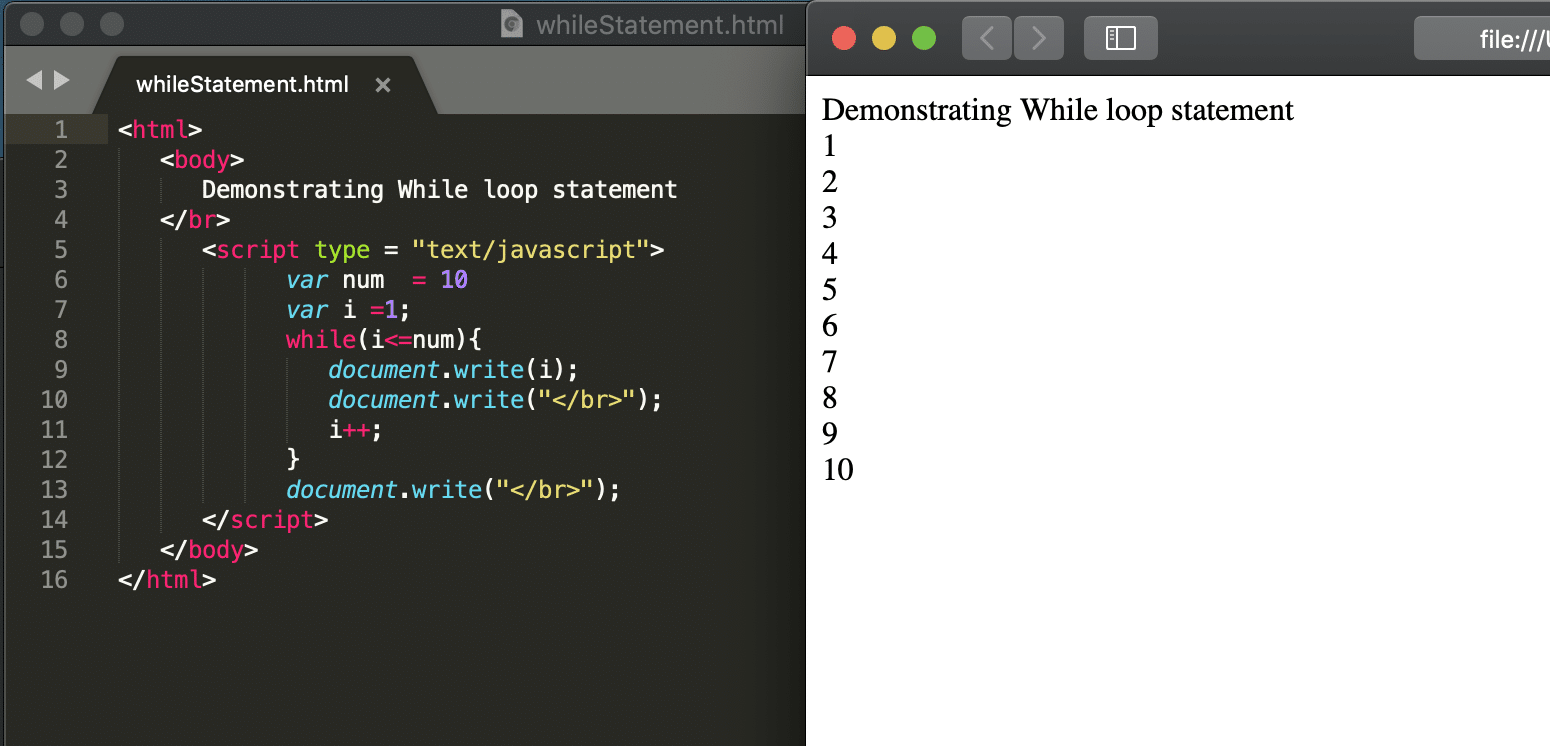As is evident from the above screenshot, the while loop initializes to index 1, and it runs until the value of index "i" reaches to 11. After each iteration, the value of index "i" is incremented by one using statement "i++" and then again compared with the condition "i<=num", which will evaluate to true for values of "i" from 1 to 10 but will evaluate to false, once the value of index "i" reaches 11.

Note: Similar to the "for" loop, the while loop is also an entry controlled loop, which means that first, the evaluation of the loop condition will happen, and then the iteration will start. So, if the condition fails at the start, then the loop will not execute even once.

Lets now try to understand a few of the differences between For loop and While Loop:

#### Difference Between For loop and While loop

For Loop While Loop
For loop knows in advance how many times it will loop While loop doesn’t know in advance how many time to loop
For loop has an initialization step While loop does not have an initialization step
For loop uses a “step value” or increment/decrement step While loop does not have increment/decrement step

### Do-While Loop in JavaScript

The do-while loop is similar to while except that it will execute the statements first and then check for the condition, whereas as we noted above, in a while loop, it will check the condition first, and then the statements will get executed.

#### What differentiates While and Do While loop?

So, for a do...while loop the set of statements will execute at least once, Because it is an exit-criteria loop, it checks the condition while the loop exits. However, in contrast to all the loops as mentioned above, which checks the condition before entering the loop. The following flowchart explains the "do .. while"  loop statement:Here we can see that the statements will execute until the condition is true, but the loop will run at least once. Because we check the condition after the execution of the statements. Hence, the name "exit-controlled" loop.

The "do..while" loop's syntax looks like below:

***Syntax: ***

``````do{

//Statements need to be executed

}while(condition);
``````

Let's understand the working of "do..while" loop with the help of following code snippet:

``````<html>

<body>

Demonstrating do..While loop statement

</br>

<script type = "text/javascript">

var num  = 10

var i =1;

do{

document.write(i);

document.write("</br>");

i++;

}while(i<=num);

document.write("</br>");

</script>

</body>

</html>

``````

Save the file with name doWhileStatement.html and open it in any browser (Chrome, Firefox, or IE). It should show the output as: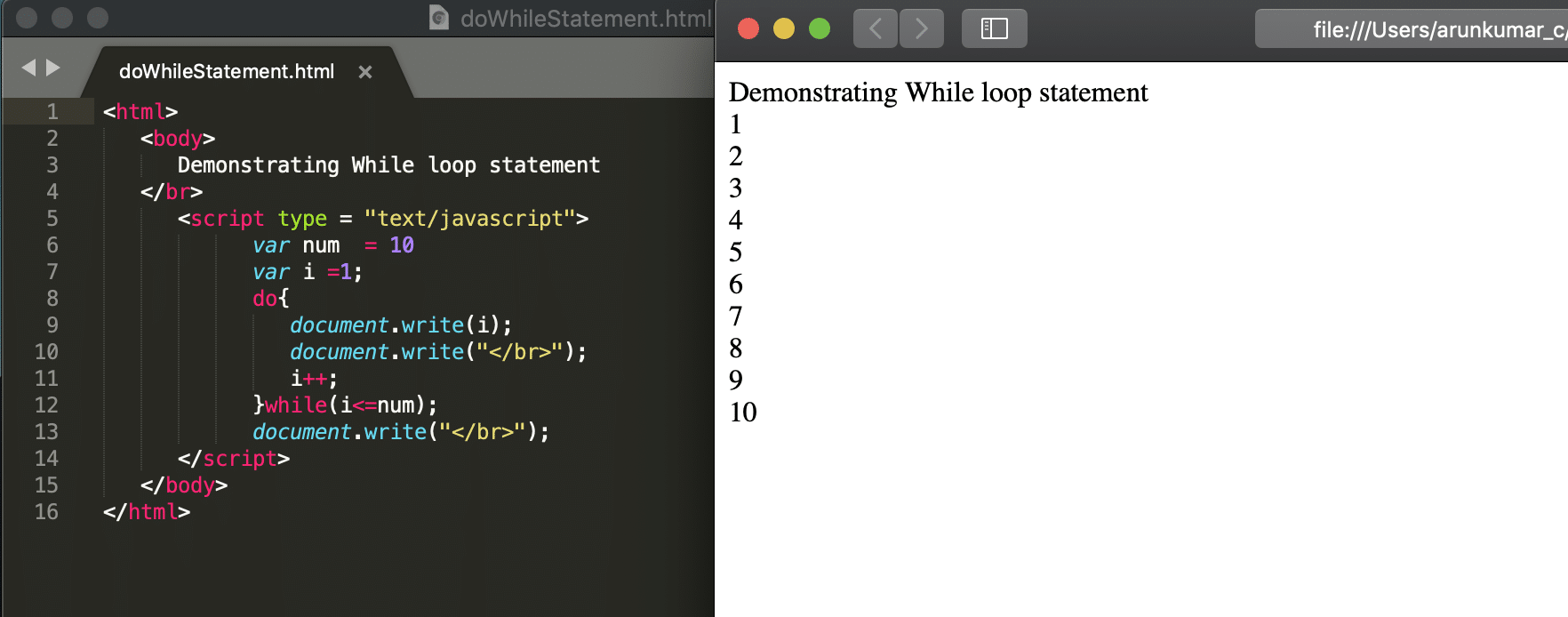As is evident from the above screenshot, the do..while first executes the statements and then compares the condition. So, even if the variable "i" would have been initialized to 11, the loop would have run at least once.

Lets now try to understand few of the differences between while and do..while loops:

#### Difference between while and Do while loop

While Loop Do While Loop
Entry-controlled loop (Checks, whether the condition specified,is valid before executing the statements in the body of the loop). It is an Exit-controlled loop (Checks the condition after executing the body of the loop).
It doesn’t execute even once if the condition is false. Executes at least once, even if the condition is false.
It is generally more useful and more commonly used. Generally less useful and much less commonly used, as compared to while loop.
Semicolon doesn't come at the end of the loop syntax. Semicolon comes at the end of the loop syntax.

## Key Takeaways

• Loops are the aids using which certain statements can iterate for a desired number of times or until a condition is true.
• JavaScript provides both entries controlled (for, while) and exit controlled (do..while) loops.
• We use For Loop when a certain logic needs to execute a certain number of times along with a condition.
• For..In and For..Of loop is used when a logic needs to be iterated based on the count of elements are present in the collection object.
• While and Do While loop is executed based on certain conditions only.

Let's move to the next article, where we learn about the concept of "Comments in JavaScript".